Next: About this document Up: My Home Page

# Introduction to Sorting Lecture 16

Steven S. Skiena

Sorting

Sorting is, without doubt, the most fundamental algorithmic problem

1. Supposedly, 25% of all CPU cycles are spent sorting
2. Sorting is fundamental to most other algorithmic problems, for example binary search.
3. Many different approaches lead to useful sorting algorithms, and these ideas can be used to solve many other problems.
What is sorting? It is the problem of taking an arbitrary permutation of n items and rearranging them into the total order,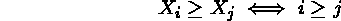Knuth, Volume 3 of ``The Art of Computer Programming is the definitive reference of sorting.

Issues in Sorting

Increasing or Decreasing Order? - The same algorithm can be used by both all we need do is change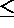to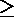in the comparison function as we desire.

What about equal keys? - Does the order matter or not? Maybe we need to sort on secondary keys, or leave in the same order as the original permutations.

What about non-numerical data? - Alphabetizing is sorting text strings, and libraries have very complicated rules concerning punctuation, etc. Is Brown-Williams before or after Brown America before or after Brown, John?

We can ignore all three of these issues by assuming a comparison function which depends on the application. Compare (a,b) should return ``<'', ``>'', or ''=''.

Applications of Sorting

One reason why sorting is so important is that once a set of items is sorted, many other problems become easy.

SearchingBinary search lets you test whether an item is in a dictionary in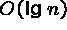time.

Speeding up searching is perhaps the most important application of sorting.

Closest pairGiven n numbers, find the pair which are closest to each other.

Once the numbers are sorted, the closest pair will be next to each other in sorted order, so an O(n) linear scan completes the job.

Element uniquenessGiven a set of n items, are they all unique or are there any duplicates?

Sort them and do a linear scan to check all adjacent pairs.

This is a special case of closest pair above.

Frequency distribution - ModeGiven a set of n items, which element occurs the largest number of times?

Sort them and do a linear scan to measure the length of all adjacent runs.

Median and SelectionWhat is the kth largest item in the set?

Once the keys are placed in sorted order in an array, the kth largest can be found in constant time by simply looking in the kth position of the array.

How do you sort?

There are several different ideas which lead to sorting algorithms:

• Insertion - putting an element in the appropriate place in a sorted list yields a larger sorted list.
• Exchange - rearrange pairs of elements which are out of order, until no such pairs remain.
• Selection - extract the largest element form the list, remove it, and repeat.
• Distribution - separate into piles based on the first letter, then sort each pile.
• Merging - Two sorted lists can be easily combined to form a sorted list.

Selection Sort

In my opinion, the most natural and easiest sorting algorithm is selection sort, where we repeatedly find the smallest element, move it to the front, then repeat...

```       * 5   7   3   2   8
2 * 7   3   5   8
2   3 * 7   5   8
2   3   5 * 7   8
2   3   5   7 * 8```

If elements are in an array, swap the first with the smallest element- thus only one array is necessary.

If elements are in a linked list, we must keep two lists, one sorted and one unsorted, and always add the new element to the back of the sorted list.

Selection Sort Implementation

```MODULE SimpleSort EXPORTS Main;  (*1.12.94. LB*)
(* Sorting and text-array by selecting the smallest element *)

TYPE
Array = ARRAY [1..N] OF TEXT;
VAR
a: Array;          (*the array in which to search*)
x: TEXT;                     (*auxiliary variable*)
last,                         (*last valid index *)
min: INTEGER;                  (* current minimum*)

BEGIN

...

FOR i:= FIRST(a) TO last - 1 DO
min:= i;                         (*index of smallest element*)
FOR j:= i + 1 TO last DO
IF Text.Compare(a[j], a[min]) = -1 THEN (*IF a[i] < a[min]*)
min:= j
END;
END; (*FOR j*)
x:= a[min];                         (* swap a[i] and a[min] *)
a[min]:= a[i];
a[i]:= x;
END; (*FOR i*)

...

END SimpleSort.```

The Complexity of Selection Sort

One interesting observation is that selection sort always takes the same time no matter what the data we give it is! Thus the best case, worst case, and average cases are all the same!

Intuitively, we make n iterations, each of which ``on average'' compares n/2, so we should make about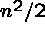comparisons to sort n items.

To do this more precisely, we can count the number of comparisons we make.

To find the largest takes (n-1) steps, to find the second largest takes (n-2) steps, to find the third largest takes (n-3) steps, ... to find the last largest takes 0 steps.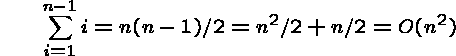An advantage of the big Oh notation is that fact that the worst case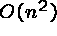time is obvious - we have n loops of at most n steps each.

If instead of time we count the number of data movements, there are n-1, since there is exactly one swap per iteration.

Insertion Sort

In insertion sort, we repeatedly add elements to a sorted subset of our data, inserting the next element in order:

```
* 5   7   3   2   8
5 * 7   3   2   8
3   5 * 7   2   8
2   3   5 * 7   8
2   3   5   7 * 8```

```

InsertionSort(A)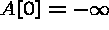for i = 1 to n-1 do

j=i

while (A[j] > A[j-1]) do swap(A[j],A[j-1])

```

In inserting the element in the sorted section, we might have to move many elements to make room for it.

If the elements are in an array, we scan from bottom to top until we find the j such that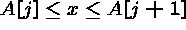, then move from j+1 to the end down one to make room.

If the elements are in a linked list, we do the sequential search until we find where the element goes, then insert the element there. No other elements need move!

Complexity of Insertion Sort

Since we do not necessarily have to scan the entire sorted section of the array, the best, worst, and average cases for insertion sort all differ!

Best case: the element always gets inserted at the end, so we don't have to move anything, and only compare against the last sorted element. We have (n-1) insertions, each with exactly one comparison and no data moves per insertion!

What is this best case permutation? It is when the array or list is already sorted! Thus insertion sort is a great algorithm when the data has previously been ordered, but slightly messed up.

Worst Case Complexity

Worst case: the element always gets inserted at the front, so all the sorted elements must be moved at each insertion. The ith insertion requires (i-1) comparisons and moves so: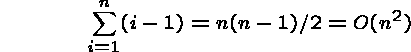What is the worst case permutation? When the array is sorted in reverse order.

This is the same number of comparisons as with selection sort, but uses more movements. The number of movements might get important if we were sorting large records.

Average Case Complexity

Average Case: If we were given a random permutation, the chances of the ith insertion requiring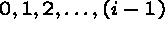comparisons are equal, and hence 1/i.

The expected number of comparisons is for the ith insertion is: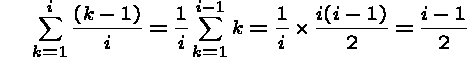Summing up over all n keys,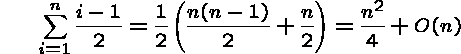So we do half as many comparisons/moves on average!

Can we use binary search to help us get belowtime?Next: About this document Up: My Home Page

Steve Skiena
Thu Oct 16 20:14:07 EDT 1997